# Power of 168 vs 618

You may learn that sets of algebraic objects has a multilinear relationship related to a vector space called tensor. Tensors may map between different objects such as vectors, scalars, even other tensors contained in a group of partitions.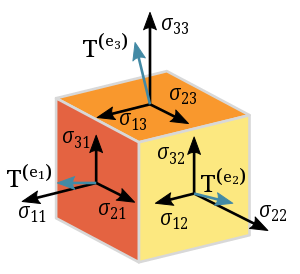In linear algebra, this vector is known as eigenvector, a nonzero vector that changes at most by a scalar factor when that linear transformation is applied to it. The corresponding eigenvalues is the factor by which the eigenvector is scaled.

A 2×2 real and symmetric matrix representing a stretching and shearing of the plane. The eigenvectors of the matrix (red lines) are the two special directions such that every point on them will just slide on them (Wikipedia).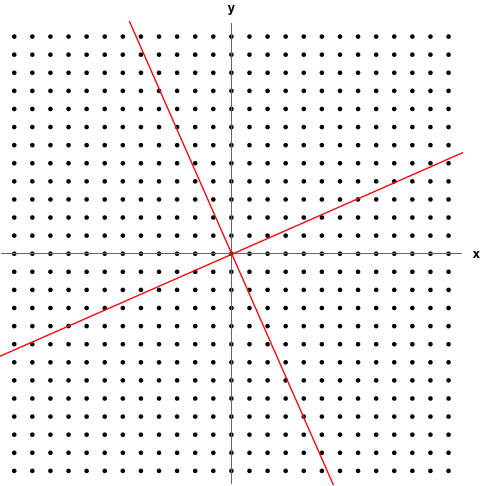The nodes are taken their place by a consideration that the 1st layer will have the same configuration as when the 3 (three) layers are combined. By this 1000 primes then 168-150=Δ18 is raised on the next turn.

String theories require extra dimensions of spacetime for their mathematical consistency. In bosonic string theory, spacetime is 26-dimensional, while in superstring theory it is 10-dimensional, and in M-theory it is 11-dimensional (Wikipedia).

``````\$True Prime Pairs:
layer | node | sub |  i   |  k   |  f
------+------+-----+------+------+-----
|      |     |  1   | 1,2  |  2,3 ← 2x = (13-11)x ---
|      |  1  +------+------+                         |
|  1   |     |  2   |  3   |  7                      |
|      |-----+------+------+                         |
|      |     |  3   | 4,6  |  10,11,12 --            |
1   +------+  2  +------+------+             |           |
|      |     |  4   |  7   |  13         5x ---      |
|      +-----+------+------+             |     |     |
|  2   |     |  5   | 8,9  |  14,15 -----      |     |
|      |  3  +------+------+                   |     |
|      |     |  6   |  10* |  19               |     |
------+------+-----+------+------+-------  lexering  |    7x + 6x = 13x
|      |     |  7   |  11* |  20               |     |
|      |  4  +------+------+                   |     |
|  3   |     |  8   |  12  |  26*  -----       |     |
|      +-----+------+------+           |       |     |
|      |     |  9   |  13  |  27       2x -----      |
2   +------|  5  +------+------+           |             |
|      |     |  10* |  14  |  28  -----              |
|      |-----+------+------+   lexering              |
|  4   |     |  11* |15,18 |  29,30,31,32 ←---4x-----
|      |  6  +------+------+
|      |     |  12  |  19  |  36
------+------+-----+------+------+-------
|      |     |  13  |  20  | {38}
|      |  7  +------+------+
|  5   |     |  14  |21,22 | {40,41} = (19-17)x -----
|      |-----+------+------+                         |
|      |     |  15  |  23  | {42}                   6x + 13x = 19x
3   +------+  8  +------+------+                         |
|      |     |  16  |24,27 | {43,44,45,46} ←- 4x ----
|      |-----+------+------+
|  6   |     |  17  |  28  | {50} = 46 + 4
|      |  9  +------+------+
|      |     |  18  |  29  | {68} = 32 + 36
------|------|-----+------+-------
``````

You may see this scheme is build by 3 (three) layers where the next layer will continue the primes object by carrying the tensor of prime 31 and 71 of previous layer. So it will return to the beginning position within 60+40=100 nodes per layer.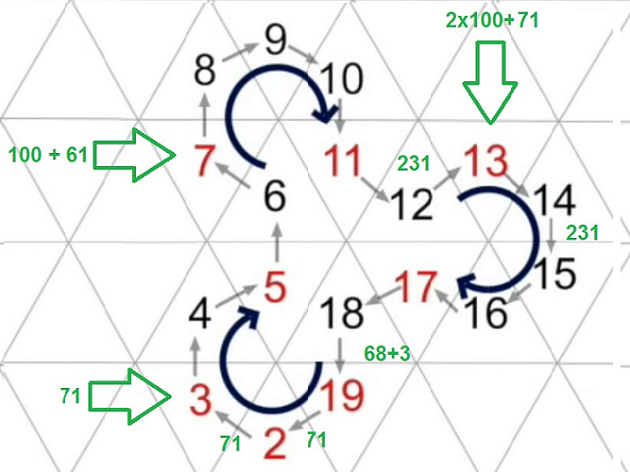It will be forced back to Δ19 making a cycle that bring back the 12 to → 13 of 9 collumns and replicate The Scheme 13:9 through (i=9,k=13)=9x3=27 with entry form of (100/50=2,60,40) as below:

``````The Prime Recycling ζ(s):
(2,3), (29,89), (36,68), (72,42), (100,50), (2,3), (29,89), ...**infinity**
----------------------+-----+-----+-----+                                    ---
7 --------- 1,2:1|   1 |  30 |  40 | 71 (2,3) ‹-------------@----        |
|                +-----+-----+-----+-----+                        |      |
|  8 ‹------  3:2|   1 |  30 |  40 |  90 | 161 (7) ‹---           |      5¨  encapsulation
|  |             +-----+-----+-----+-----+             |          |      |
|  |  6 ‹-- 4,6:3|   1 |  30 | 200 | 231 (10,11,12) ‹--|---       |      |
|  |  |          +-----+-----+-----+-----+             |   |      |     ---
--|--|-----» 7:4|   1 |  30 |  40 | 200 | 271 (13) --›    | {5®} |      |
|  |          +-----+-----+-----+-----+                 |      |      |
--|---› 8,9:5|   1 |  30 | 200 | 231 (14,15) ---------›       |      7¨  abstraction
289        |          +-----+-----+-----+-----+-----+                  |      |
|          ---› 10*:6|  20 |   5 |  10 |  70 |  90 | 195 (19) --› Φ   | {6®} |
--------------------+-----+-----+-----+-----+-----+ lexering         |     ---
67 -------› 11*:7|   5 |   9 |  14 (20) --------› ¤               |      |
|                +-----+-----+-----+                              |      |
|  78 ‹----- 12:8|   9 |  60 |  40 | 109 (26) «------------       |     11¨  polymorphism
|  |             +-----+-----+-----+                       |      |      |
|  |  86‹--- 13:9|   9 |  60 |  69 (27) «-- Δ19 (Rep Fork) | {2®} |      |
|  |  |          +-----+-----+-----+                       |      |     ---
|  |   --› 14:10*|   9 |  60 |  40 | 109 (28) -------------       |      |
|  |             +-----+-----+-----+ lexering                     |      |
|   --› 15,18:11*|   1 |  30 |  40 | 71 (29,30,31,32) ------------      13¨  inheritance
329  |                +-----+-----+-----+                                     |
|   ‹--------- 19:12|  10 |  60 | {70} (36) ‹--------------------- Φ        |
-------------------+-----+-----+                                          ---
786 ‹------- 20:13|  90 |  90 (38) ‹-------------- ¤                      |
|                +-----+-----+                                           |
| 618 ‹- 21,22:14|   8 |  40 |  48 (40,41) ‹----------------------      17¨  class
|  |             +-----+-----+-----+-----+-----+                  |      |
|  | 594 ‹- 23:15|   8 |  40 |  70 |  60 | 100 | 278 (42) «--     |{6'®} |
|  |  |          +-----+-----+-----+-----+-----+             |    |     ---
--|--|-»24,27:16|   8 |  40 |  48 (43,44,45,46) ------------|----       |
|  |          +-----+-----+                               |           |
--|---› 28:17| 100 | {100} (50) ------------------------»           19¨  object
168        |          +-----+                                                 |
|         102 -› 29:18| 50  | 50(68) ---------> Δ18                           |
----------------------+-----+                                                ---

Faktors:
168 = 12x14 = 8x21 = 7x24 = 6x28 = 4x42 = 3x56 = 2x84
618 = 6x103 = 6x(100+3) = 3x206 = 3x(200+6) = 2x309 = 2x(300+9)
1+6+8 = π(1x6x8) = π(1x48) = π(2x24) = π(3x16)= π(4x12) = π(6x8)

Permutations:
168 = 102 + 66 = 2x3x((8+9)+(2+9)) = π(Φ(289+329)) = π(Φ((8+9)²+(1+2)&29))
168 + 618 = 168 + 329 + 289 = (7x24) + (7x47) + (8+9)² = (7x71) + (17x17)

- By 168 this will be 329+30+30-160 or 229 which is the 50th primes so it span 50
- This 229 carry 1000 to 1229 = π(10000) means it is touching the end of 329 layer
- So the 229 converted to 1000 - 50 or 950 which is 950/10+1 or 96 on the 168 layer
``````

In the second opposing member, the position 13 in the second term gives a redundant value of the template 7 of 7 × 7 = 49. The opposite prime position 31 as the 11th prime number is now forced to determine a new axis-symmetrical zero position.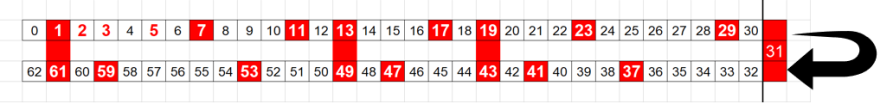By this project we are going to use a library called Chevrotain. It can be used to build Lexers, Parsers and Interpreters for various use cases ranging from simple config files to full fledged programming languages.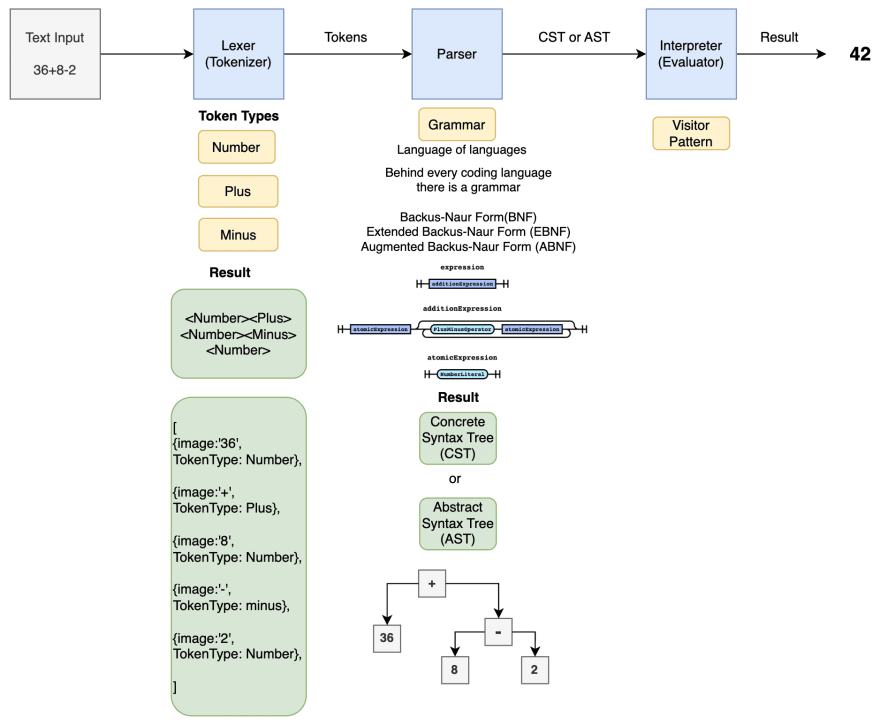Each result goes to the 9th object of prime 67 which is 19th prime. So when the cycle has passed the 10th object then the 43 objects will be laid by 9 collumns and slightly forming a recombination which facilitate them to finaly generate 1000 primes